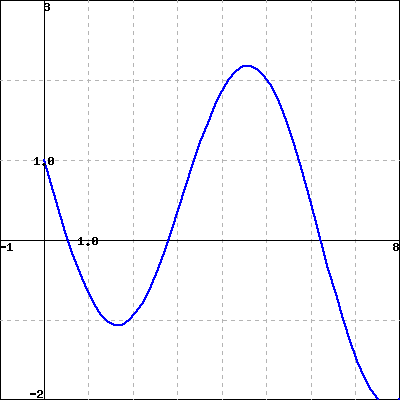Let $g(x)=\int_{3}^{x}\, f(t)\,dt$, where $f(t)$ is given in the figure below.(Click on the graph for a larger version.)

Find each of the following:

A. $g(3) =$

B. $g'(6) =$

C. The interval (with endpoints given to the nearest 0.25) where $g$ is concave up:
interval =
(Give your answer as an interval or a list of intervals, e.g., (-infinity,8] or (1,5),(7,10), or enter none for no intervals.)

D. The value of $x$ where $g$ takes its maximum on the interval $0 \leq x \leq 8$.
$x =$